# A search for deeplybound kaonic nuclear states by

• Slides: 21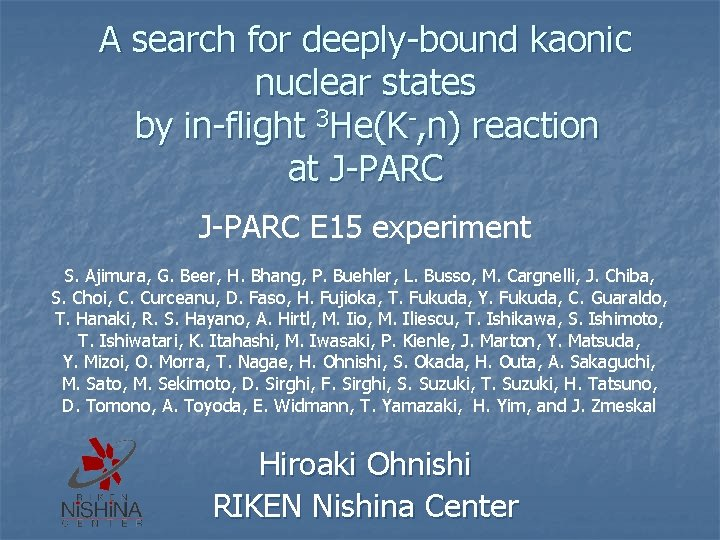A search for deeply-bound kaonic nuclear states by in-flight 3 He(K-, n) reaction at J-PARC E 15 experiment S. Ajimura, G. Beer, H. Bhang, P. Buehler, L. Busso, M. Cargnelli, J. Chiba, S. Choi, C. Curceanu, D. Faso, H. Fujioka, T. Fukuda, Y. Fukuda, C. Guaraldo, T. Hanaki, R. S. Hayano, A. Hirtl, M. Iio, M. Iliescu, T. Ishikawa, S. Ishimoto, T. Ishiwatari, K. Itahashi, M. Iwasaki, P. Kienle, J. Marton, Y. Matsuda, Y. Mizoi, O. Morra, T. Nagae, H. Ohnishi, S. Okada, H. Outa, A. Sakaguchi, M. Sato, M. Sekimoto, D. Sirghi, F. Sirghi, S. Suzuki, T. Suzuki, H. Tatsuno, D. Tomono, A. Toyoda, E. Widmann, T. Yamazaki, H. Yim, and J. Zmeskal Hiroaki Ohnishi RIKEN Nishina Center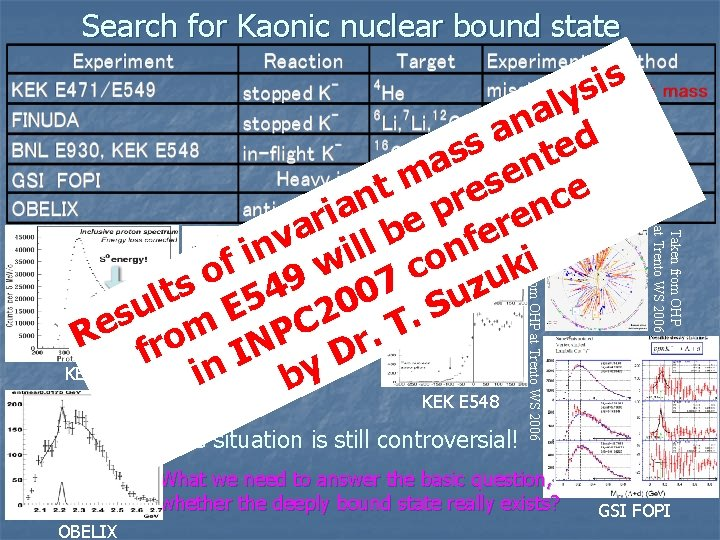Search for Kaonic nuclear bound state What we need to answer the basic question, whether the deeply bound state really exists? OBELIX Taken from OHP at Trento WS 2006 The situation is still controversial! Taken from OHP at Trento WS 2006 Phys. Rev. Lett. 94 212303 (2005) s i s y l a n a ed s s t a n e m s t e e n c r a e p ren i r a lb e v f l n n i i i o f w k c o 49 u 7 s z lt E 5 200 Su u s m. C e T. R fro INP r D KEK E 549 in FINUDAby KEK E 548 GSI FOPI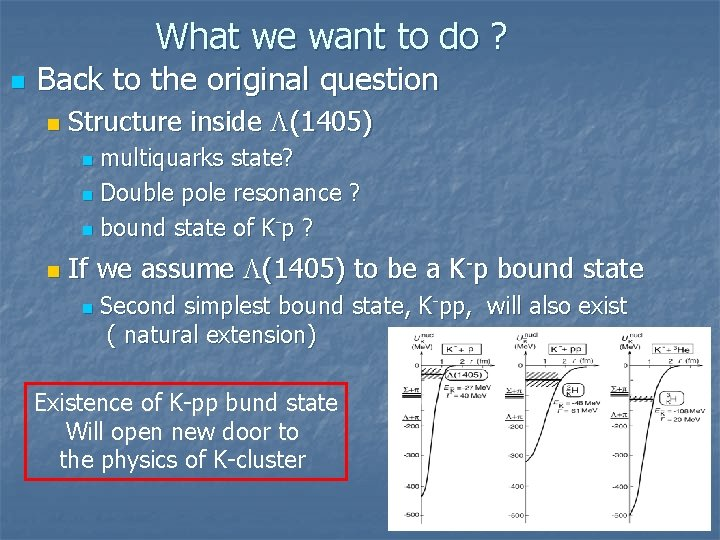What we want to do ? n Back to the original question n Structure inside L(1405) multiquarks state? n Double pole resonance ? n bound state of K-p ? n n If we assume L(1405) to be a K-p bound state n Second simplest bound state, K-pp, will also exist ( natural extension) Existence of K-pp bund state Will open new door to the physics of K-clusterA search for deeply bound kaonic nuclear states Goal for the experiment J-PARC E 15 n To answering the basic question : whether the deeply bound state really exists? n Direct production of K-pp clusters via 3 He(in-flight K-, n) reaction n K- 3 He Reaction ? ? ? K-pp cluster Decay neutron ss a m y g n cop i s s Mi ctros on r e t p u S e n via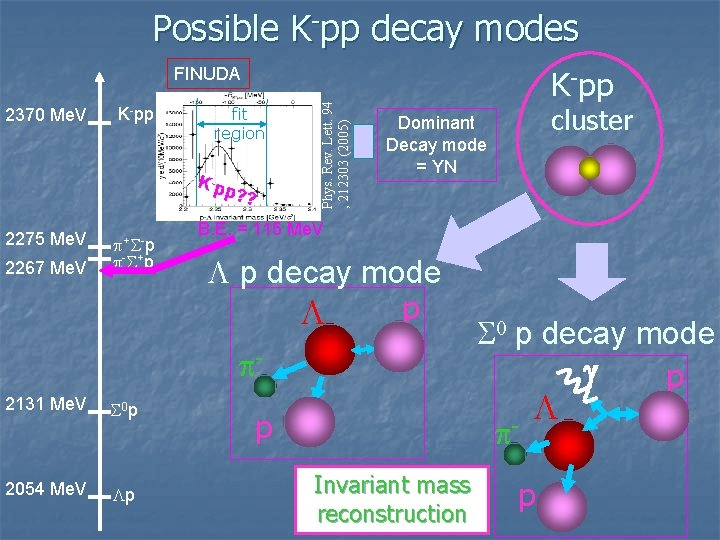Possible K-pp decay modes 2370 Me. V K-pp fit region K -pp ? ? 2275 Me. V 2267 Me. V p+ S- p p- S+ p Phys. Rev. Lett. 94 , 212303 (2005) FINUDA K-pp B. E. = 115 Me. V L p decay mode p L p- 2131 Me. V S 0 p 2054 Me. V Lp cluster Dominant Decay mode = YN p S 0 p decay mode g p p- Invariant mass reconstruction L p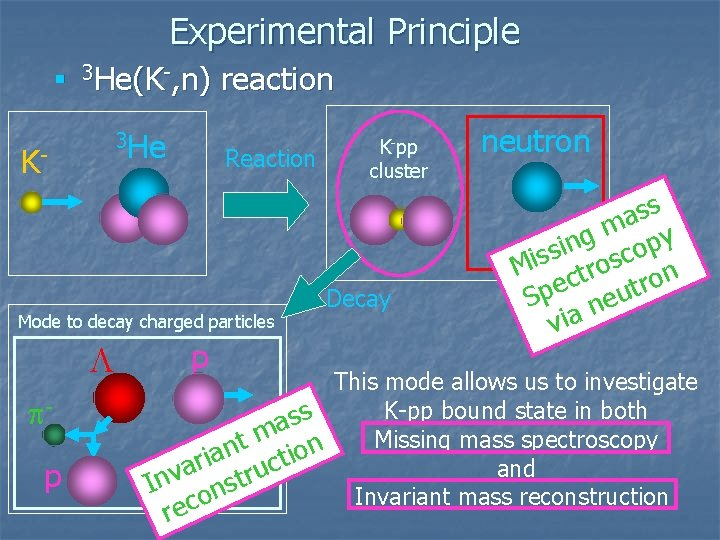Experimental Principle n 3 He(K-, n) 3 He K- reaction Reaction Mode to decay charged particles L pp p K-pp cluster Decay neutron ss a m y g n cop i s s Mi ctros on r e t p u S e n via This mode allows us to investigate K-pp bound state in both s s a m Missing mass spectroscopy t n n o a i i t r c and a tru v n s I n Invariant mass reconstruction o c re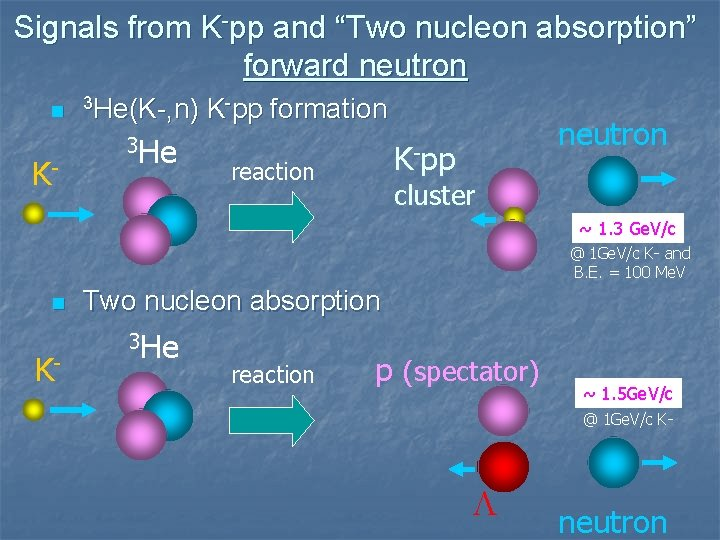Signals from K-pp and “Two nucleon absorption” forward neutron n　3 He(K-, n) K- 3 He K-pp formation reaction neutron K-pp cluster ~ 1. 3 Ge. V/c @ 1 Ge. V/c K- and B. E. = 100 Me. V n　Two K- nucleon absorption　 3 He reaction p (spectator) ~ 1. 5 Ge. V/c @ 1 Ge. V/c K- L neutronHow was decay side? n　3 He(K-, n) K- 3 He Binding energy assumed as １００ＭｅＶ => emitted proton momentum ～ 500 Me. V/c K-pp formation reaction p neutron K-pp L cluster - p n　Two K- p nucleon absorption　 3 He reaction (spectator) pp(spectator) p. Major background, i. e. event from “two nucleon absorption process” can be identified! p L L neutronDesign for the experimentBeam Line at J-PARC Beam Spectrometer FF Q 8 Primary Proton beam n ES K 1. 8 BR Beam Line n n n Design for low momentum Kaon (<1. 2 Ge. V/c ) Single ES stage Beam line length = 27. 6 m QDQ to focus at Final Focus (FF) Momentum resolution ~ 2 x 10 -4 (position resolution of the beam tracker assumed to be 200 mm (s) ) D 4 Q 7E 15 Detector concept Experiment will be performed at K 1. 8 BR beam line. Neutron counter Cylindrical detector system H D C GEANT 4 detector simulation Proton K- p- B. E. = 100 Me. V t e gn C D C So o len i a M d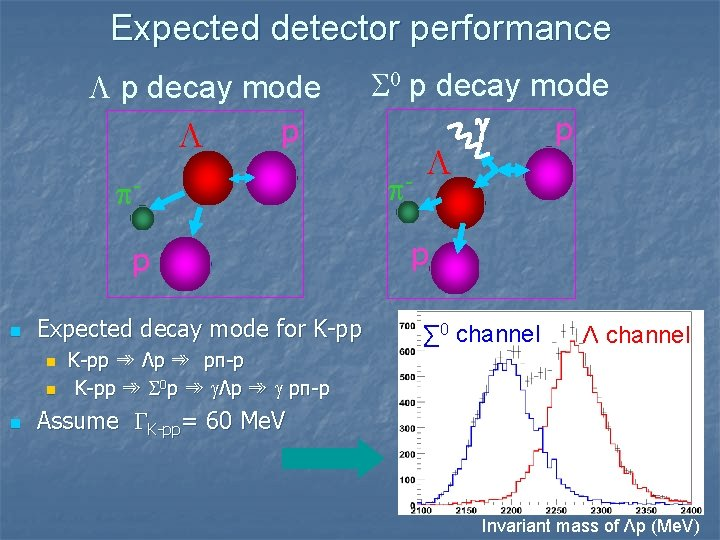Expected detector performance L p decay mode p L pp n Expected decay mode for K-pp n n n K-pp ➾ Λp ➾ pπ-p K-pp ➾ S 0 p ➾ gΛp ➾ g pπ-p S 0 p decay mode g p p- L p ∑ 0 channel Λ channel Assume GK-pp= 60 Me. V　　 Invariant mass of Λp (Me. V)Status of the preparationNeutron counter n We will use same neutron counter used for KEK E 549 experiment ( exist! ) E 549 neutron counter 3. 2 m 1. 5 m 20 x 5 x 150 cm 3　（WＸTＸL） Plastic scintillator　 Configuration 16 (wide)　x　7 (depth) Surface area 3. 2 m X 1. 5 mSolenoid magnet for E 15 experiment has been constructed. Field strength; upto 0. 7 T Space inside; Φ=1. 2 m, L=1. 2 m weight ; 23 tCylindrical Drift Chamber Design work for CDC is in progress Basic concept ; • Cell structure has been chosen as Hexagonal • 15 readout layers AA’A UU’ VV’ AA’ UU’ VV’ AA • 1800 readout channels in total • He based chamber gas will be used (to reduce material budget) Garfield simulation done by Dr. F. Sakuma n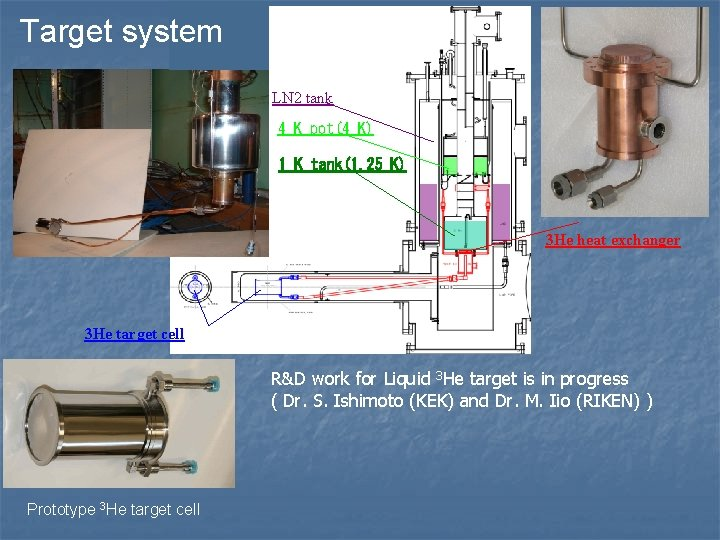Target system LN 2 tank 4 K pot(4 K) 1 K tank(1. 25 K) 3 He heat exchanger 3 He target cell R&D work for Liquid 3 He target is in progress ( Dr. S. Ishimoto (KEK) and Dr. M. Iio (RIKEN) ) Prototype 3 He target cell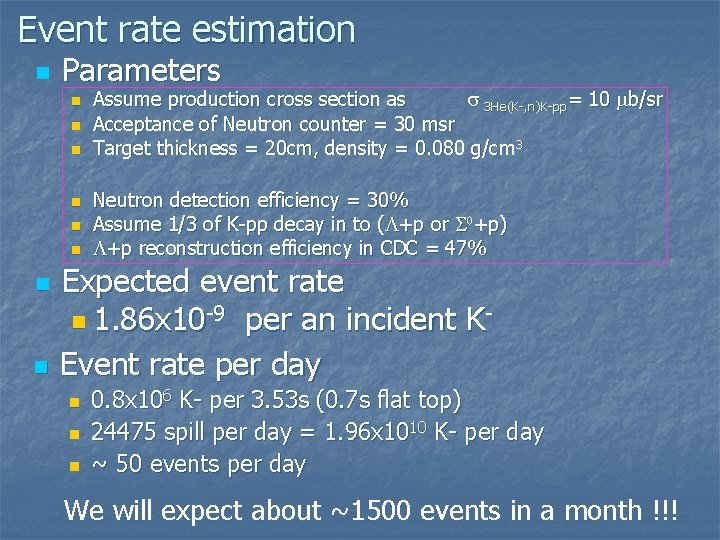Event rate estimation n Parameters n n n n Assume production cross section as s 3 He(K-, n)K-pp= 10 mb/sr Acceptance of Neutron counter = 30 msr Target thickness = 20 cm, density = 0. 080 g/cm 3 Neutron detection efficiency = 30% Assume 1/3 of K-pp decay in to (L+p or S 0+p) L+p reconstruction efficiency in CDC = 47% Expected event rate n 1. 86 x 10 -9 per an incident KEvent rate per day n n n 0. 8 x 106 K- per 3. 53 s (0. 7 s flat top) 24475 spill per day = 1. 96 x 1010 K- per day ~ 50 events per day We will expect about ~1500 events in a month !!!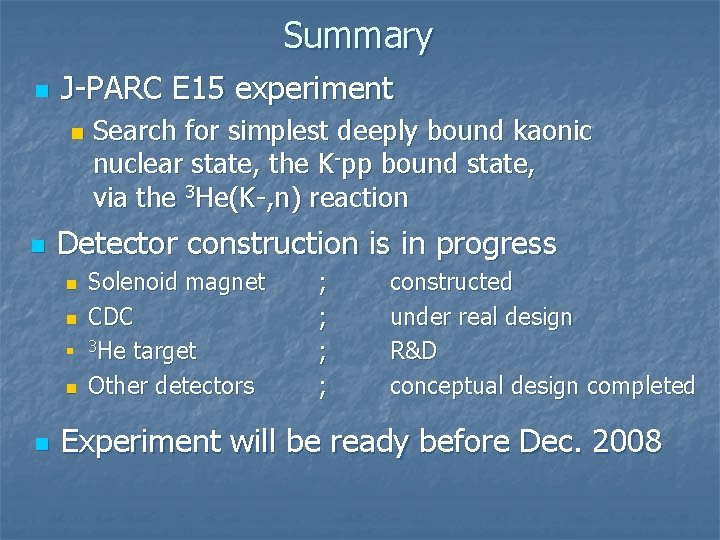Summary n J-PARC E 15 experiment n n Detector construction is in progress n n n Search for simplest deeply bound kaonic nuclear state, the K-pp bound state, via the 3 He(K-, n) reaction Solenoid magnet CDC 3 He target Other detectors ; ; constructed under real design R&D conceptual design completed Experiment will be ready before Dec. 2008Thank you very much